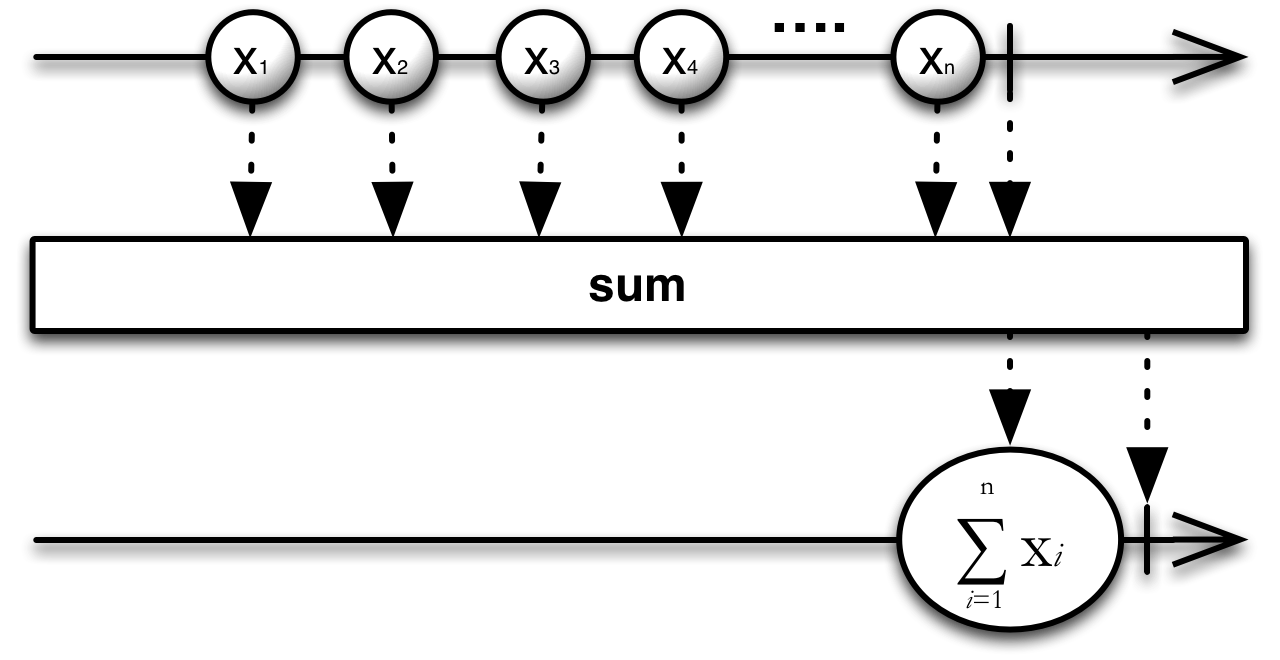# Sum¶

• Official ReactiveX documentation: Sum
Observable.sum(key_selector=None)

Computes the sum of a sequence of values that are obtained by invoking an optional transform function on each element of the input sequence, else if not specified computes the sum on each item in the sequence.

Example res = source.sum() res = source.sum(lambda x: x.value)

key_selector – {Function} [Optional] A transform function to apply to
each element.

Returns an observable {Observable} sequence containing a single element with the sum of the values in the source sequence.